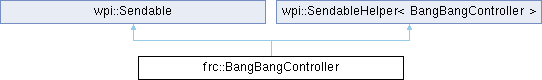WPILibC++ 2023.4.3
frc::BangBangController Class Reference

Implements a bang-bang controller, which outputs either 0 or 1 depending on whether the measurement is less than the setpoint. More...

#include <frc/controller/BangBangController.h>

Inheritance diagram for frc::BangBangController:## Public Member Functions

BangBangController (double tolerance=std::numeric_limits< double >::infinity())
Creates a new bang-bang controller. More...

void SetSetpoint (double setpoint)
Sets the setpoint for the bang-bang controller. More...

double GetSetpoint () const
Returns the current setpoint of the bang-bang controller. More...

bool AtSetpoint () const
Returns true if the error is within the tolerance of the setpoint. More...

void SetTolerance (double tolerance)
Sets the error within which AtSetpoint will return true. More...

double GetTolerance () const
Returns the current tolerance of the controller. More...

double GetMeasurement () const
Returns the current measurement of the process variable. More...

double GetError () const
Returns the current error. More...

double Calculate (double measurement, double setpoint)
Returns the calculated control output. More...

double Calculate (double measurement)
Returns the calculated control output. More...

void InitSendable (wpi::SendableBuilder &builder) override
Initializes this Sendable object. More...Public Member Functions inherited from wpi::Sendable
virtual ~Sendable ()=default

virtual void InitSendable (SendableBuilder &builder)=0
Initializes this Sendable object. More...Public Member Functions inherited from wpi::SendableHelper< BangBangController >
SendableHelper (const SendableHelper &rhs)=default

SendableHelper (SendableHelper &&rhs)

SendableHelperoperator= (const SendableHelper &rhs)=default

SendableHelperoperator= (SendableHelper &&rhs)Protected Member Functions inherited from wpi::SendableHelper< BangBangController >
SendableHelper ()=default

~SendableHelper ()

## Detailed Description

Implements a bang-bang controller, which outputs either 0 or 1 depending on whether the measurement is less than the setpoint.

This maximally-aggressive control approach works very well for velocity control of high-inertia mechanisms, and poorly on most other things.

Note that this is an asymmetric bang-bang controller - it will not exert any control effort in the reverse direction (e.g. it won't try to slow down an over-speeding shooter wheel). This asymmetry is extremely important. Bang-bang control is extremely simple, but also potentially hazardous. Always ensure that your motor controllers are set to "coast" before attempting to control them with a bang-bang controller.

## ◆ BangBangController()

 frc::BangBangController::BangBangController ( double tolerance = std::numeric_limits< double >::infinity() )
explicit

Creates a new bang-bang controller.

Always ensure that your motor controllers are set to "coast" before attempting to control them with a bang-bang controller.

Parameters
 tolerance Tolerance for atSetpoint.

## ◆ AtSetpoint()

 bool frc::BangBangController::AtSetpoint ( ) const

Returns true if the error is within the tolerance of the setpoint.

Returns
Whether the error is within the acceptable bounds.

## ◆ Calculate() [1/2]

 double frc::BangBangController::Calculate ( double measurement )

Returns the calculated control output.

Parameters
 measurement The most recent measurement of the process variable.
Returns
The calculated motor output (0 or 1).

## ◆ Calculate() [2/2]

 double frc::BangBangController::Calculate ( double measurement, double setpoint )

Returns the calculated control output.

Always ensure that your motor controllers are set to "coast" before attempting to control them with a bang-bang controller.

Parameters
 measurement The most recent measurement of the process variable. setpoint The setpoint for the process variable.
Returns
The calculated motor output (0 or 1).

## ◆ GetError()

 double frc::BangBangController::GetError ( ) const

Returns the current error.

Returns
The current error.

## ◆ GetMeasurement()

 double frc::BangBangController::GetMeasurement ( ) const

Returns the current measurement of the process variable.

Returns
The current measurement of the process variable.

## ◆ GetSetpoint()

 double frc::BangBangController::GetSetpoint ( ) const

Returns the current setpoint of the bang-bang controller.

Returns
The current setpoint.

## ◆ GetTolerance()

 double frc::BangBangController::GetTolerance ( ) const

Returns the current tolerance of the controller.

Returns
The current tolerance.

## ◆ InitSendable()

 void frc::BangBangController::InitSendable ( wpi::SendableBuilder & builder )
overridevirtual

Initializes this Sendable object.

Parameters
 builder sendable builder

Implements wpi::Sendable.

## ◆ SetSetpoint()

 void frc::BangBangController::SetSetpoint ( double setpoint )

Sets the setpoint for the bang-bang controller.

Parameters
 setpoint The desired setpoint.

## ◆ SetTolerance()

 void frc::BangBangController::SetTolerance ( double tolerance )

Sets the error within which AtSetpoint will return true.

Parameters
 tolerance Position error which is tolerable.

The documentation for this class was generated from the following file: